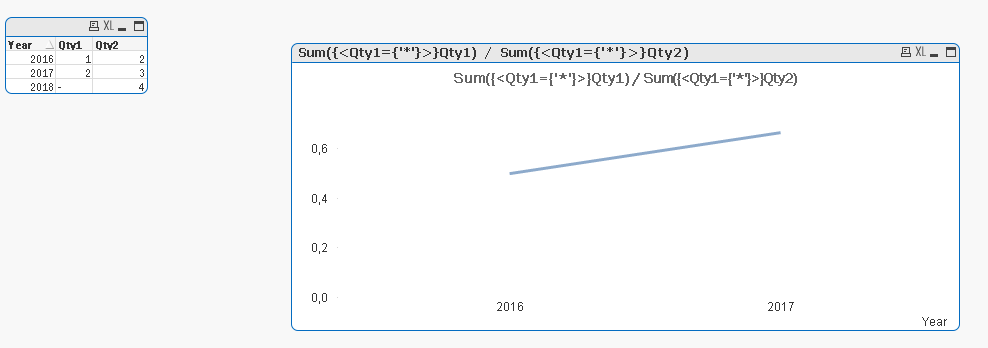Qlik Sense App Development

Highlighted
New Contributor II

Exclude value in dimension using set analysis

Data Sample:

YearSum (Qty 1)Sum (Qty 2)

2016

12
201723
2018NULL4

Formula: Sum (Qty 1)/Sum(Qty 2)

Problem: I'm trying to create a line graph of Year vs [Sum( Qty 1) / Sum( Qty 2)]. The graph looks weird because it drops to 0 in 2018 due to the NULL value in Sum (Qty 1). Is there anyway to get the graph to not display year 2018 because Sum (Qty 1) is Null? How would you code that in set expression?

Any help would be greatly appreciated!!

Tags (1)
1 Solution

Accepted Solutions
New Contributor II

Re: Exclude value in dimension using set analysis

Hi Jason,

You can also use : Sum({<Qty1={'*'}>}Qty1) / Sum({<Qty1={'*'}>}Qty2) in order to avoid Null values,

Regards

Jon5 RepliesMVP

Re: Exclude value in dimension using set analysis

May be this

Sum({<Year -= {2018}>}Qty 1)/Sum({<Year -= {2018}>}Qty 2)

New Contributor II

Re: Exclude value in dimension using set analysis

Hey Sunny, thanks for the response. That was my first thought, but I was hoping there would be a way that I could avoid hardcoding a specific year to ignoreMVP

Re: Exclude value in dimension using set analysis

May be this

Sum({<Year = {"=Sum(Qty 1) > 0"}>}Qty 1)/Sum({<Year = {"=Sum(Qty 1) > 0"}>}Qty 2)

New Contributor II

Re: Exclude value in dimension using set analysis

Hi Jason,

You can also use : Sum({<Qty1={'*'}>}Qty1) / Sum({<Qty1={'*'}>}Qty2) in order to avoid Null values,

Jon